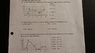# Acceleration, Velocity and Position

• cvc121
So for interval C to D the acceleration is positive. For question 3, since the entire graph is above the x-axis in the positive area, wouldn't the acceleration between each of the intervals be positive with the one exception where it is zero?No. Graph 2 is velocity. Any time velocity is increasing (it doesn't matter if it is always positive), the acceleration is positive. It doesn't matter if velocity is always positive. Any time velocity is decreasing, the acceleration is negative.Understood.

## Homework Statement

Can anyone verify if I am interpreting and converting the different graphs correctly? I believe that I am on the right track but would really appreciate some confirmation. Thanks! All help is very much appreciated!

N/A

## The Attempt at a Solution

The questions as well as my solutions are attached below.

#### Attachments

•20160429_215227.jpg
30.7 KB · Views: 371
It looks like you might know how to do it but should double check your answers. There are a couple of mistakes. If you describe your thinking that leads to your answers, we can tell if you understand it.

Thanks for your reply. For question 1a), all I thought about were the tangents at each of those locations. If the slope of the tangent was positive, the instantaneous velocity was also positive. If it was negative, the instantaneous velocity would also be negative. For question 1b), I just thought about the speed and direction of the object. For instance, slowing down in a forward direction would result in a negative acceleration while speeding up in the backwards direction would also be negative acceleration. For interval C to D since the object is moving backwards at a constant velocity and slows down just at the end, would the acceleration be positive (a little bit confused about this one)? For questions 3, since the entire graph is above the x-axis in the positive area, wouldn't the acceleration between each of the intervals be positive with the one exception where it is zero? If anyone could point out where I went wrong it would be very much appreciated since interval C to D for question 1b) is the only one that I am unsure of. Thanks!

I would just like to add that I believe that for question #2 interval B to C and C to D that the accelerations are negative, not positive since they are slowing down in the forward direction.

cvc121 said:
I would just like to add that I believe that for question #2 interval B to C and C to D that the accelerations are negative, not positive since they are slowing down in the forward direction.
Check 1b) C to D
cvc121 said:
Thanks for your reply. For question 1a), all I thought about were the tangents at each of those locations. If the slope of the tangent was positive, the instantaneous velocity was also positive. If it was negative, the instantaneous velocity would also be negative. For question 1b), I just thought about the speed and direction of the object. For instance, slowing down in a forward direction would result in a negative acceleration while speeding up in the backwards direction would also be negative acceleration. For interval C to D since the object is moving backwards at a constant velocity and slows down just at the end, would the acceleration be positive (a little bit confused about this one)?
You correctly say that the velocity is negative at C and zero at D. So what does that say about the acceleration? I would only think about the ends C & D and not worry about in between.

For questions 3, since the entire graph is above the x-axis in the positive area, wouldn't the acceleration between each of the intervals be positive with the one exception where it is zero?
No. Graph 2 is velocity. Any time velocity is increasing (it doesn't matter if it is always positive), the acceleration is positive. It doesn't matter if velocity is always positive. Any time velocity is decreasing, the acceleration is negative.

Ok. Understood. So for 1b) interval C to D is positive acceleration since the object is slowing down in the backwards direction?

cvc121 said:
Ok. Understood. So for 1b) interval C to D is positive acceleration since the object is slowing down in the backwards direction?
From your answers in 1a), the velocity is negative at C and zero at D. That is an increase in velocity from C to D. That means the acceleration is positive.